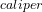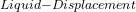## A student experimentally determines the density of a plastic cube using the caliper method, liquid displacement method, and suspension metho

Question

A student experimentally determines the density of a plastic cube using the caliper method, liquid displacement method, and suspension method. The cube’s true density is 0.9822 g/cm3. Use the student’s collected data below to answer the following questions.

Student’s Collected Data

Cube’s Mass 0.66g
Caliper Method
Edge Length 0.85 cm
Liquid Displacement Method
Volume of Liquid 5.5 mL
Volume of Liquid + Object 6.2 mL

Required:
Determine the density of the cube for each method.

in progress 0
2 months 2021-07-29T19:31:00+00:00 1 Answers 8 views 0

density of the cube for Caliper Method is 1.08 g/mL

density of the cube for Liquid Displacement Method is 0.94 g/mL

Explanation:

Given the data in the question;

The cube’s true density = 0.9822 g/cm³

Student’s Collected Data;

Cube’s Mass = 0.66g

Caliper Method

Edge Length = 0.85 cm

Liquid Displacement Method

Volume of Liquid = 5.5 mL

Volume of Liquid + Object = 6.2 mL

For Caliper Method;

Edge Length = 0.85 cm

so the volume of the cube will be ( 0.85 cm )³ OR 0.614125 cm³ ≈ 0.61 cm³ = 0.61 mL

so Density will be;

Density= mass of cube / volume of cube

we substitute

Density= 0.66g / 0.61 mL

Density= 1.08 g/mL

Therefore, density of the cube for Caliper Method is 1.08 g/mL

For Liquid Displacement Method;

Volume of object = total volume – volume of liquid

= 6.2 mL – 5.5 mL = 0.7 mL

now, Density of object will be;

Density= mass / volume

we substitute

Density= 0.66 g / 0.7 mL

Density= 0.942857 ≈  0.94 g/mL

Therefore, density of the cube for Liquid Displacement Method is 0.94 g/mL